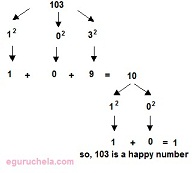# Happy / Sad Number Calculation

The calculator determins the given number is a Happy Number or Sad Number.

 Enter Positive Integer:

 Result

### List of few happy numbers (between 1 and 1000)

1, 7, 13, 19, 23, 28, 44, 49, 68, 79, 129, 133, 139, 167, 188, 226, 236, 239, 338, 356, 367, 368, 379, 446, 469, 478, 556, 566, 888, 899.

### List of few un-happy numbers (between 1 and 20)

2, 3, 4, 5, 6, 8, 9, 11, 12, 14, 15, 16, 17, 18, 20

The famous female mathematician (Sophie Germain), discovered that all numbers can be categorized as “happy” or “unhappy.” She concluded, a happy number is one in which the sum of each digit squared eventually ends in the number 1.

In recreational mathematics, the "happy numbers" and "sad numbers" are the integers that produce particular sequences when you repeatedly square the number's digits and compute the sum of those squares. Integers that stabilize at 1 are called "happy numbers" and integers that fall into the loop are called "sad numbers”. For the example, Positive Integer = 103

Sum of Digits of 103 = 1² + 0² + 3² = 1 + 0 + 9 = 10

10 = 1² + 0² = 1 + 0 = 1

so 103 is a happy number